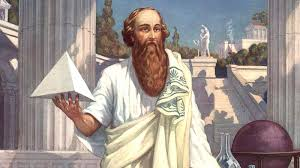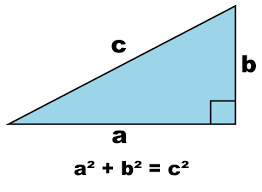# Pythagoras:Pythagoras:

Pythagoras of Samos was a famous Greek mathematician and philosopher (c. 570 – c. 495 BC).

He is known best for the proof of the important Pythagorean theorem.

Which is about right angle triangles.
He started a group of mathematicians, called the Pythagoreans.

Pythagoras was born in Samos, a little island off the western coast of Asia Minor. Pythagoras theorem.

Pythagoras is most famous for his theorem to do with right triangles.He said that the length of the hypotenuse (C) squared would be equal to the sum of the squares of lengths of the other two sides. And so (a x a) + (b x b) = (c x c) was born.

Wishing you all the best,
http://www.seeyourneeds.in DONATE AS YOU LIKE